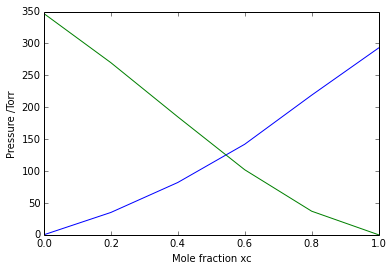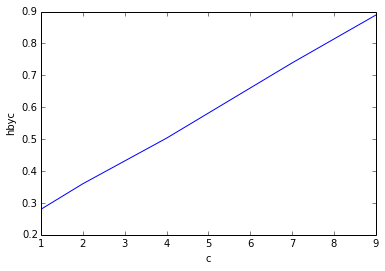# Chapter 6 - The properties of mixtures¶

## Example E1 - Pg 112¶

In :
#Calculate the mole fraction of glycerine
#Initialization of variables
m=0.14 #mol/kg
w=1. #kg Assume
#Calculations
ngly=m*w
nwater=w*1000 /18.02
ntotal=ngly+nwater
xgly=ngly/ntotal
#results
print '%s %.2e' %('Mole fraction of glycerine  is xgly =',xgly)

Mole fraction of glycerine  is xgly = 2.52e-03


## Example E2 - Pg 114¶

In :
#calculate the volume of the mixture
#Initialization of variables
mE=50. #g
mW=50. #g
#calculations
nE=mE/46.
nW=mW/18.
ntotal=nE+nW
xE=nE/ntotal
xW=1-xE
print '%s' %('for the observed xE and xW')
vE=55 #cc/mol
vW=18 #cc/mol
V=nE*vE+nW*vW
#results
print '%s %.1f %s' %('\n Volume of the mixture =',V,'cm^3 ')

for the observed xE and xW

Volume of the mixture = 109.8 cm^3


## Example E3 - Pg 121¶

In :
# Calculate Ka and Kc from the raoults law line
#Initialization of variables
import matplotlib
from matplotlib import pyplot
xc=([0, 0.20, 0.40, 0.60, 0.80, 1])
pc=([0, 35, 82, 142, 219, 293])
pa=([347, 270, 185, 102, 37, 0])
#calculations
pyplot.plot(xc,pc)
pyplot.plot(xc,pa)
pyplot.xlabel('Mole fraction xc')
pyplot.ylabel('Pressure /Torr')
print '%s' %('From the graph it is clear that KA=175 torr and KC=165 torr. They are plotted with Raoults law lines')
pyplot.show()

From the graph it is clear that KA=175 torr and KC=165 torr. They are plotted with Raoults law lines## Example E4 - Pg 123¶

In :
#Calculate if required concentration can be maintained under normal conditions.
#Initialization of variables
import math
C=4/1000. #g/L
MO2=32. #g/mol
Mw=18.
w=1 #L
K=3.3*math.pow(10,7) #torr
patm=0.21*760 #torr
#calculations
nO2=C/MO2
nH2O=w*1000. /Mw
xO2=nO2/(nO2+nH2O)
pO2=xO2*K
if(pO2<patm):
print '%s %.1e' %('The required concentration can be maintained under normal conditions and pressure is (Torr)',pO2)
else:
print '%s %.e' %('The required concentration cannot be maintained under normal conditions and pressure is (Torr)',pO2)

The required concentration can be maintained under normal conditions and pressure is (Torr) 7.4e+01


## Example E5 - Pg 131¶

In :
#Calculate the molar mass of the enzyme
#Initialization of variables
%matplotlib inline
import numpy as np
from numpy import linalg
import matplotlib
from matplotlib import pyplot
c=([1, 2, 4, 7, 9])
hbyc=([0.28, 0.36, 0.503, 0.739, 0.889])
R=8.3145 #J/K mol
T=298 #K
g=9.81 #m/s^2
d=0.9998 #g/cm^3
#calculations
pyplot.plot(c,hbyc)
pyplot.xlabel('c')
pyplot.ylabel('hbyc')
A = np.vstack([c, np.ones(len(c))]).T
vector=numpy.linalg.lstsq(A,hbyc)
intercept=vector
intercept1=intercept/100.
M=R*T/(d*g*intercept1)/1000.
#results
print '%s %d %s' %('Molar mass of the enzyme is close to',M,'kDa')
print '%s' %('The answer is a bit different in the textbook due to rounding off error in the textbook')
pyplot.show()

Populating the interactive namespace from numpy and matplotlib
Molar mass of the enzyme is close to 123 kDa
The answer is a bit different in the textbook due to rounding off error in the textbook## Example E6 - Pg 136¶

In :
#calculate how many times the rich phase is more abundant in nitrobenzene
#Initialization of variables
nB=0.59 #mol
nNB=0.41 #mol
xN1=0.38
xN2=0.74
xNm=0.41
#calculations
print '%s' %('By lever rule')
ratio=(xNm-xN1)/(xN2-xNm)
percent=ratio*100. +1
#results
print '%s %d %s' %("The rich phase is",percent,"times more abundant in nitrobenzene")

By lever rule
The rich phase is 10 times more abundant in nitrobenzene